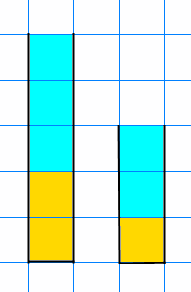#### You may also like### Tweedle Dum and Tweedle Dee

Two brothers were left some money, amounting to an exact number of pounds, to divide between them. DEE undertook the division. "But your heap is larger than mine!" cried DUM...### Sum Equals Product

The sum of the numbers 4 and 1 [1/3] is the same as the product of 4 and 1 [1/3]; that is to say 4 + 1 [1/3] = 4 ï¿½ 1 [1/3]. What other numbers have the sum equal to the product and can this be so for any whole numbers?### Special Sums and Products

Find some examples of pairs of numbers such that their sum is a factor of their product. eg. 4 + 12 = 16 and 4 × 12 = 48 and 16 is a factor of 48.

##### Age 11 to 14Challenge LevelI mixed up some lemonade in two glasses.

The first glass had $200$ml of lemon juice and $300$ml of water.
The second glass had $100$ml of lemon juice and $200$ml of water.

Which mixture has the stronger tasting lemonade?
How do you know?

Use the interactivity below to compare different mixtures of lemonade and develop a strategy for deciding which is stronger each time.

Click the New Mixes button to generate new mixtures

Beaker A

Lemon ???
Water ???

Beaker B

Lemon ???
Water ???

Which beaker has the stronger tasting lemonade?

Score: 0

Once you are confident that you can always work out which mixture is stronger, here are some questions to consider:

How might you use fractions to help you to work out which mixture is stronger?
How might you use ratios?